Latest Banking jobs   »

# Reasoning Quizzes Quiz For IRDA AM 2023-10th May

Direction (1-5): Read the following information carefully to give the answers to the questions.
In a certain code language,
‘One step solution racism’ is coded as ‘ba sa ea ca’
‘Racism demand deal for’ is coded as ‘xa fa ma ca’
‘Close demand up been’ is coded as ‘na da ma ga’
‘Mid close for step’ is coded as ‘ga fa ba ya’

Q1. What is the code of ‘demand’?
(a) xa
(b) ga
(c) da
(d) ma
(e) None of the above

Q2. Which is the following word is coded as ‘ya’?
(a) Mid
(b) Been
(c) One
(d) Race
(e) None of the above

Q3. What is the code of ‘racism solution’?
(a) ca ea
(b) da na
(c) ca sa
(d) ma xa
(e) Either (a) or (c)

Q4. Which is the following word is coded as ‘fa’?
(a) One
(b) For
(c) Demand
(d) Deal
(e) None of the above

Q5. What is the code of ‘deal’?
(a) ya
(b) da
(c) na
(d) xa
(e) None of the above

Q6. If it is possible to make a five-letter meaningful word from 4th, 5th, 8th, 9th & 10th letters from the left end of the of word “DOCUMENTARY”(each letter is used only once), then which will be the 3rd letter of that meaningful word? If no meaningful word is formed, then mark the answer as X. If more than one meaningful word is formed then, mark the answer as Z.
(a) A
(b) T
(c) Z
(d) R
(e) X

Q7. If it is possible to make a five-letter meaningful word from 1st,7th, 8th, 9th & 11th letters from the left end of the of word “PROSECUTION” (each letter is used only once), then which will be the last letter of that meaningful word? If no meaningful word is formed, then mark the answer as X. If more than one meaningful word is formed then, mark the answer as Z.
(a) N
(b) T
(c) U
(d) Z
(e) X

Q8. How many pair of letters are there in the word “BAHNSCHRIFT” which has as many letters between them as in the alphabetical series (both forward and backward direction)?
(a) One
(b) Two
(c) Three
(d) Four
(e) None of the above

Q9. How many pair of letters are there in the word “SEMICONDENSED” which has as many letters between them as in the alphabetical series (both forward and backward direction)?
(a) One
(b) Two
(c) Three
(d) Four
(e) None of the above

Q10. How many pair of letters are there in the word “CAMBRIA” which has as many letters between them in the word as in the alphabetical series (both forward and backward direction)?
(a) None
(b) Two
(c) Three
(d) Four
(e) One

Directions (11-15): In each of the questions below, some statements are given followed by two conclusions. You have to take the given statements to be true even if they seem to be at variance with commonly known facts. Read all the conclusions and then decide which of the given conclusions logically follows from the given statements, disregarding commonly known facts. Give answer

Q11. Statements: Some breeze is thunder.
Some rain is cloud.
No rain is thunder
Conclusions: I. No cloud is thunder.
II. Some breeze is cloud is a possibility.
(a) Both I and II follow
(b) Either I or II follows
(c) Only II follows
(d) Only I follows
(e) Neither I nor II follows

Q12. Statements: All blue is black.
Some black is not white.
No white is grey.
Conclusions: I. Some black being blue is a possibility
II. Some black is grey.
(a) Both I and II follow
(b) Either I or II follows
(c) Only II follows
(d) Only I follows
(e) Neither I nor II follows

Q13. Statements: Some Tree is Soil.
All Soil is Plant.
All Plant is Water
Conclusions: I. Some Tree is Plant.
II. Some Tree is not Plant.
(a) Both I and II follow
(b) Either I or II follows
(c) Only II follows
(d) Only I follows
(e) Neither I nor II follows

Q14. Statements: All universe is star.
Some star is earth.
No earth is galaxy
Conclusions: I. All galaxy is universe
II. Some galaxy is not universe.
(a) Both I and II follow
(b) Either I or II follows
(c) Only II follows
(d) Only I follows
(e) Neither I nor II follows

Q15. Statements: Some coffee is milk.
No milk is tea.
Some tea is water
Conclusions: I. Some water can be milk.
II. Some coffee can be tea.
(a) Both I and II follow
(b) Either I or II follows
(c) Only II follows.
(d) Only I follows.
(e) Neither I nor II follows

Solutions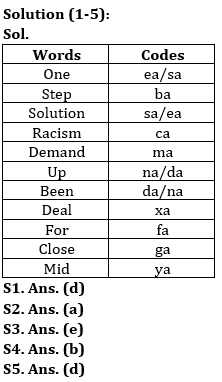S6. Ans. (e)
Sol. 4th, 5th, 8th, 9th & 10th letters of the given word are U, M, T, A & R. So, no meaningful word is formed.

S7. Ans. (b)
Sol. 1st,7th, 8th, 9th & 11th letters of the given word are P, U, T, I & N. So, the word formed is INPUT. Hence, last letter will be T.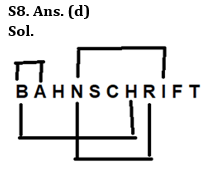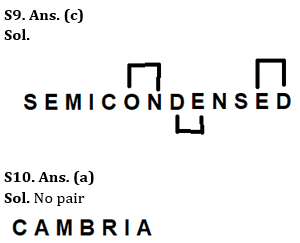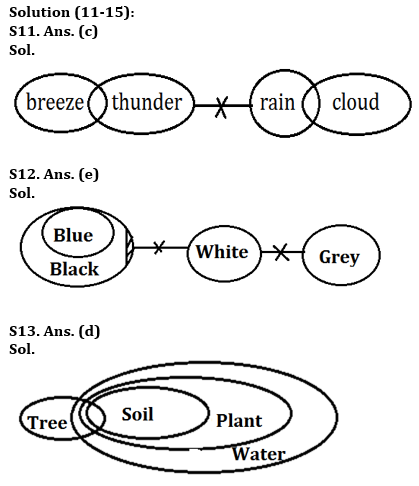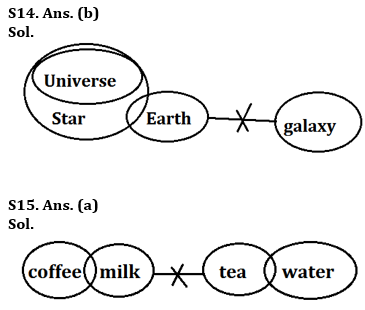## FAQs

### How many sections are there in the IRDAI exam?

There are 4 sections in the IRDAI assistant manager online preliminary exam. They are Reasoning, English Language, General awareness, Quantitative aptitude.

#### Congratulations!Union Budget 2023-24: Free PDF